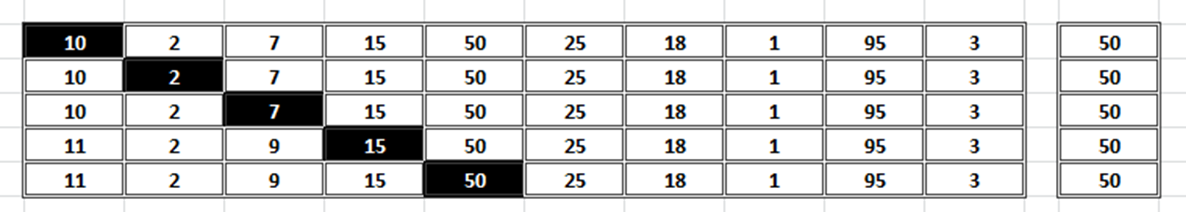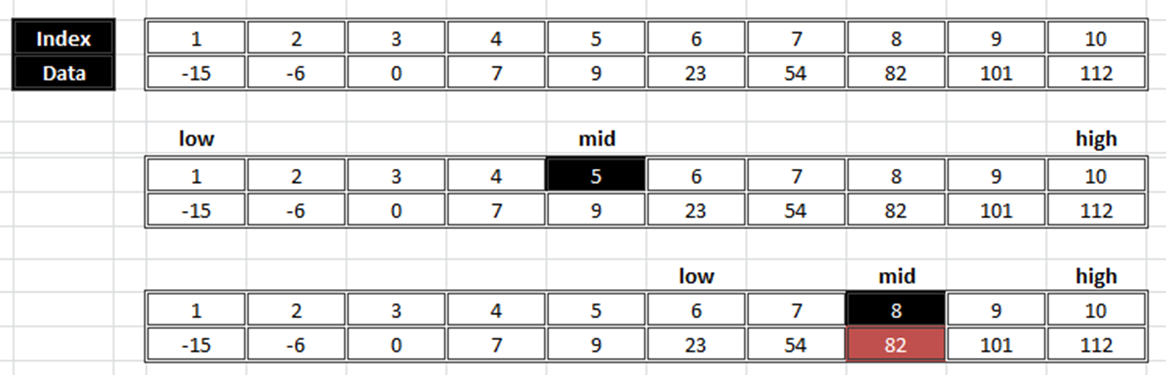• My previous post described some useful string handling functions to deal with string data for different operations. In this post I am about to explain one of the most common and frequently required operation called Searching.
• Searching is most common operation that is required to perform on the set of data. We often need to look for a particular peach of data of our interest in the available set of data. If data is large enough then manual searching might be time consuming and in some cases it might not be feasible at all.
• Today’s majority of the software and devices whether it is our smartphone where we search for particular contact or messages, email or social media sites supports searching operation as it is the primary requirement to deal with the data for better understanding and analysis. Search engines like google, yahoo are the best examples of how searching helps us to make our life more comfortable. Although they uses more complex algorithms, they works on the same principle.
• In this post, I will show you how the searching can be implemented using C programming language.

Searching is a process to find out some element from the given set of data.

• The search operation may be successful if search value is found and unsuccessful if not found.
• There are two standard search methods:
1. Linear search
2. Binary search.

### Linear search

• Linear Search is the simplest searching method.
• In this method the search element is sequentially searched in the list.
• It can be applied to a sorted or unsorted list of data.
• In case of sorted list, searching starts from 0th element and continues until the element is found or a greater value is found [assume list is sorted in ascending order].
• In case of unsorted list, searching starts from 0th element and continues until the element is found or up to the end of the list.
##### Example:
• Now consider the following example of unsorted array of 10 elements.• Suppose searching element is 50. So 50 is compared with all the elements starting from 0th element.
• The searching process is over either 50 is found or the list is over.

#### Linear Search Implementation

``````// C Program to implement linear Search.
#include "stdio.h"

void main()
{
int array, n, i, search, found = 0;

printf("Enter No.of elements (Maximum 50):");
scanf("%d",&n);
printf("Enter %d integers values...\n", n);

for (i = 0; i < n; i++)
scanf("%d",&array[i]);

printf("Enter value to Serch :");
scanf("%d", &search);
for (i = 0; i < n; i++)
{
if ( search == array[i] )
{
found = 1;
printf("%d found at location %d.\n", search, i+1);
break;
}

}
if ( found == 0 )

getch();
}
``````
• Notice that we have used flag variable called “found” just to keep track about the failure of search operation by setting it with initial value 0.
• We are setting value 1 if the search value is found and let the user notify by showing the message. If the search value is not present in the list, flag variable found  will be unchanged so outside the for loop we are checking against its initial value so that we can notify about the failure of the search operation.

### Binary Search

• Another most common searching technique is the binary search.
• It uses divide and conquer technique for searching.
• Binary search can only be applied on sorted data. That means data should be in sorted order before applying binary search.
• According to this technique an array or list of data is divided into two parts from some mid point by using the following formula:
``mid = (low+high) / 2;``
• where, mid = mid point , low = starting array index and high= last index of an array.
• After splitting an array or list into two parts, there are three possibilities:
1. Search value > array[mid]:  In this case search value is greater than the array             [mid] value so searching will be done in the right direction.
2. Search value < array[mid]: In this case search value is less than the mid point value so searching will be carried on the left side.
3. Search = array[mid] : In this case our searching is finished as we found the desired value.
##### Example:

Consider the following example to find out element 82 in the given set of elements:• An array contains 10 elements so initially low  will set to index 1 and high will be set to index 10 (note: array index always tarts from 0 but for simplicity we have assume 1).
• Since our array has more than two elements, first it will be divided into two sub parts from the mid point using the formula:
``````mid = (low+ high) / 2

mid = (1+10) / 2

mid = 5 ;``````
• In the first iteration array will be divided from the 5th index as shown in the second figure.
• Since our search value 82 is greater than the value present at mid point which is 9, search will be done in the right direction with modified low value (low =mid +1: e.i. 5+1 = 6).
• Again the right sub array will be divided into the two sub parts using the same concept.
``````mid = ( 6+10) /2

mid = 8``````
• We have new mid value as 8 in the net iteration and this time the third case will be executed and so we will get our search value 82 as our mid is equal to our search value.
• Binary search offers major improvement in the performance than the linear search.

#### Binary Search Implementation

``````// C Program to implement Binary Search
#include "stdio.h"

void main()
{
int array, i, low, high, mid, n, search;

printf("Enter No.of elements (Maximum 50):");
scanf("%d",&n);
printf("Enter %d integers values...\n", n);

for (i = 0; i < n; i++)
scanf("%d",&array[i]);

printf("Enter value to Serch :");
scanf("%d", &search);

low = 0;
high = n - 1;
mid = (low+high)/2;

while (low <= high)
{
if (array[mid] < search) // search in right direction
low = mid + 1;
else if (array[mid] == search) // if mid is the search value
{
printf("%d Found at location %d.\n", search, mid+1);
break;
}
else    // search in left direction
high = mid - 1;

mid = (low + high)/2; // split into two parts
}

if (low > high)